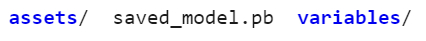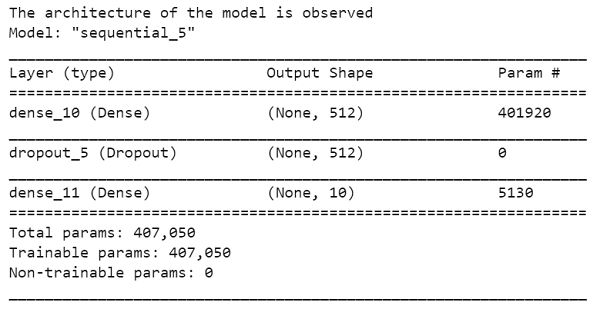# How can Keras be used to reload a fresh model from the saved model using Python?

Tensorflow is a machine learning framework that is provided by Google. It is an open−source framework used in conjunction with Python to implement algorithms, deep learning applications and much more. It is used in research and for production purposes.

The ‘tensorflow’ package can be installed on Windows using the below line of code −

pip install tensorflow

Tensor is a data structure used in TensorFlow. It helps connect edges in a flow diagram. This flow diagram is known as the ‘Data flow graph’. Tensors are nothing but multidimensional array or a list. They can be identified using three main attributes −

• Rank − It tells about the dimensionality of the tensor. It can be understood as the order of the tensor or the number of dimensions in the tensor that has been defined.

• Type − It tells about the data type associated with the elements of the Tensor. It can be a one dimensional, two dimensional or n dimensional tensor.

• Shape − It is the number of rows and columns together.

Keras means ‘horn’ in Greek. Keras was developed as a part of research for the project ONEIROS (Open ended Neuro-Electronic Intelligent Robot Operating System). It runs on top of Tensorflow framework. It was built to help experiment in a quick manner. It is highly scalable, and comes with cross platform abilities. This means Keras can be run on TPU or clusters of GPUs. Keras models can also be exported to run in a web browser or a mobile phone as well.

Keras is already present within the Tensorflow package. It can be accessed using the below line of code.

import tensorflow
from tensorflow import keras

We are using the Google Colaboratory to run the below code. Google Colab or Colaboratory helps run Python code over the browser and requires zero configuration and free access to GPUs (Graphical Processing Units). Colaboratory has been built on top of Jupyter Notebook. Following is the code −

## Example

ls saved_model/my_model
print("The architecture of the model is observed")
new_model.summary()

## Output## Explanation

• The newly saved model is displayed on the console.

• The new model is loaded and stored in a variable.

• The details associated with the new model is displayed on the console using the ‘summary’ method.